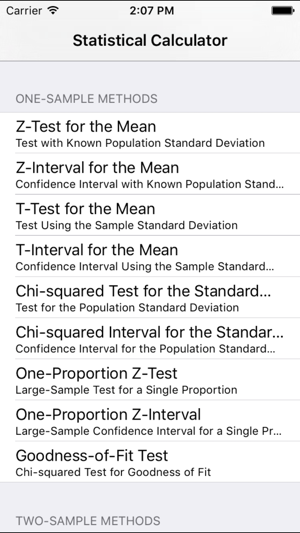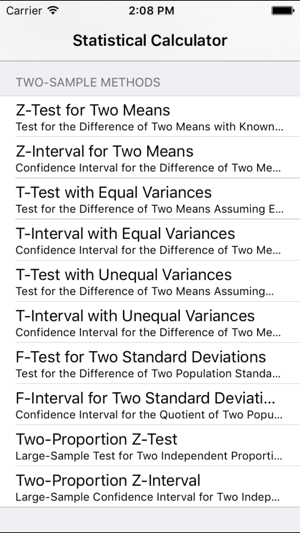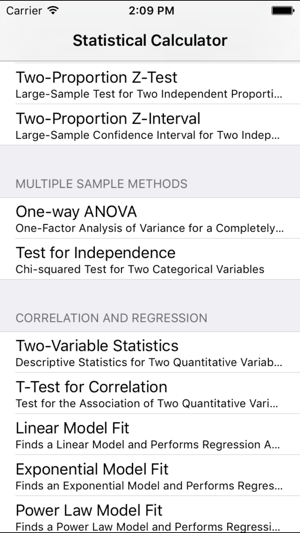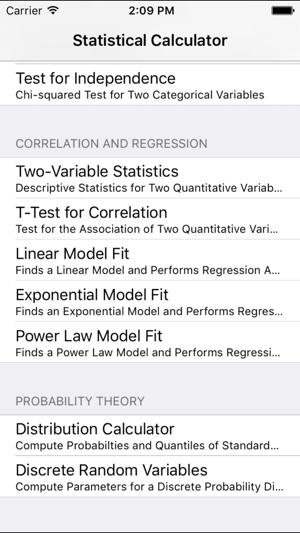## iPhone Screenshots

•••••## Description

Short Description:

Computes descriptive statistics of quantitative variables.
Performs statistical tests and computes confidence intervals in one, two and multiple-sample situations.
Provides regression analysis using linear, exponential and power-law models.
Computes probabilities and quantiles for standard statistical distributions.
Provides statistics features usually found on hand-held calculators.
Accuracy of numerical results tested against Wolfram Mathematica.

Features:

Data Entry:
Easy (list-based) entry of quantitative and categorical data, and two-way tables.
Voice-based data entry (Siri) is possible.

One-Sample Methods:
Z-Test for the Mean.
Z-Interval for the Mean.
T-Test for the Mean.
T-Interval for the Mean.
Wilcoxon Signed Rank Test.
Chi-squared Test for the Standard Deviation.
Chi-squared Interval for the Standard Deviation.
One-Proportion Z-Test.
One-Proportion Z-Interval.
Chi-squared Test for Goodness-of-Fit.

Two-Sample Methods:
Z-Test for Two Means.
Z-Interval for Two Means.
T-Test for for Two Means (Assuming Equal Variances).
T-Interval for Two Means (Assuming Equal Variances).
T-Test for for Two Means (Assuming Unequal Variances).
T-Interval for Two Means (Assuming Unequal Variances).
F-Test for for Two Standard Deviations.
F-Interval for Two Standard Deviations.
Two-Proportion Z-Test.
Two-Proportion Z-Interval.
McNemar Test.

Multiple Sample Methods:
One-way ANOVA.
Chi-squared Test for Independence.
Cochran's Q-Test

Correlation and Regression:
Two-Variable Descriptive Statistics.
T-Test for Correlation.
Linear Model Fit and Regression Analysis.
Exponential Model Fit and Regression Analysis.
Power-Law Model Fit and Regression Analysis.
Spearman Rank Correlation.

Probability Theory:
Distribution Calculator: Contains methods for computing probabilities and quantiles for standard statistical distributions.
Computes Mean, Variance and Standard Deviation for a Discrete Random Variable.

## What’s New

Version 1.5

Added Cochran's Q-Test.

## Information

Seller
Ulrich Hoensch
Size
3.2 MB
Category
Education
Compatibility

Requires iOS 9.0 or later. Compatible with iPhone, iPad, and iPod touch.

Languages

English

Age Rating
Rated 4+
Copyright
© 2017 Ulrich Hoensch
Price
Free

## Supports

•